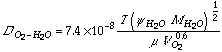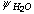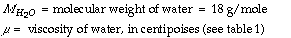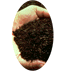## Calculating the Oxygen Diffusion Coefficient in Water

### Tom Richard

This discussion is part of a section on oxygen transport and oxygen diffusion in compost, which provides background on the general concepts and equations.

Estimates of the diffusion coefficient in liquids often use a correlation developed by Wilke and Chang, 1955, which is based on the Stokes-Einstein equation:where= an "association" parameter for the solvent water = 2.26 (Reid et al., 1977)= the molar volume of oxygen = 25.6 cm3/g - mole (Welty et al., 1984)

This equation, which lacks the rigorous theoretical foundation used to develop the equations for gas mixtures, is usually accurate to ± 10% for dilute solutions of nondissociating solutes (Bird, et al., 1960).

For the purposes of this analysis, we neglect the effects of dissolved substrate and microorganisms and assume that the solvent is water. Substituting the given values into this equation, we can calculate the diffusion constant for oxygen in an aqueous solution. The results of this calculation, for the range of temperatures common in composting systems, are provided in Table 1 (Calculating the Oxygen Diffusion Coefficient in Air).

Table 2.

Effect of Temperature and on the Oxygen Diffusion Coefficients in Water

Temperature Viscosity of H2O
[centipoises]
(Weast, 1969)
O2 Diffusion Coefficient
[cm2/s]
20°C 1.002 0.0000197
40°C 0.653 0.0000324
50°C 0.547 0.0000399
60°C 0.467 0.0000482

From these results we can see that the oxygen diffusion coefficient in water is strongly affected by temperature. This effect is even stronger than was the case in air, more than doubling as temperatures increase from 20°C to 60°C.

The other, more significant, comparison with the oxygen diffusion coefficient calculated in air is the magnitude of the coefficient. The O2 diffusion coefficient in saturated air (15% oxygen) is 5,700 to 10,800 times greater than in water (60°C and 20°C respectively). When oxygen is forced to diffuse through water saturated pores, this restriction on oxygen transport quickly leads to anaerobic conditions.

These implications are discussed in further in the section on factors leading to anaerobic conditions.

References:

Bird, R.B., W.E. Stewart, and E.N. Lightfoot. 1960. Transport Phenomena. John Wiley & Sons. NY. 780 pp. Return to citation in text.

Reid, R.C., J.M. Praunsnitz, and T.K. Sherwood. 1977. The Properties of Gases and Liquids, Third Edition. McGraw-Hill. NY. p. 578. Return to citation in text.

Weast, R.C. (ed.) 1969. CRC Handbook of Chemistry and Physics, 50th Edition. p F-36. Return to citation in text.

Welty, J.R., C.E. Wicks, and R.E. Wilson. 1984. Fundamentals of Momentum, Heat, and Mass Transfer, Third Edition. John Wiley & Sons,NY. 803 pp. Return to citation in text.

Wilke, C.R., and P. Chang. 1955. A.E.CH.E. Journal 1: 264-270. Return to citation in text.Cornell Composting Science & Engineering Composting in Schools Resources